## Example Questions

### Example Question #51 : Integers

If x represents an even integer, which of the following expressions represents an odd integer?

2x – 2

3x – 2

3x + 1

5x + 4

x + 2

3x + 1

Explanation:

Pick any even integer (2, 4, 6, etc.) to represent x. The only value that is odd is 3x + 1. Any number multiplied by an even integer will be even. When an even number is added and subtracted to that product, the result will be even as well. 3x + 1 is the only choice that adds an odd number to the product.

### Example Question #11 : Integers

Add: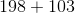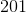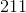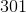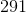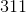Explanation: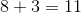Since the number is 10 or greater, the tens digit is the carryover.

Add the tens digit with the carryover.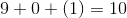Add the hundreds digit with the carryover.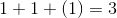Combine all the ones digits in our calculations.

The answer is.

### All SAT Math Resources